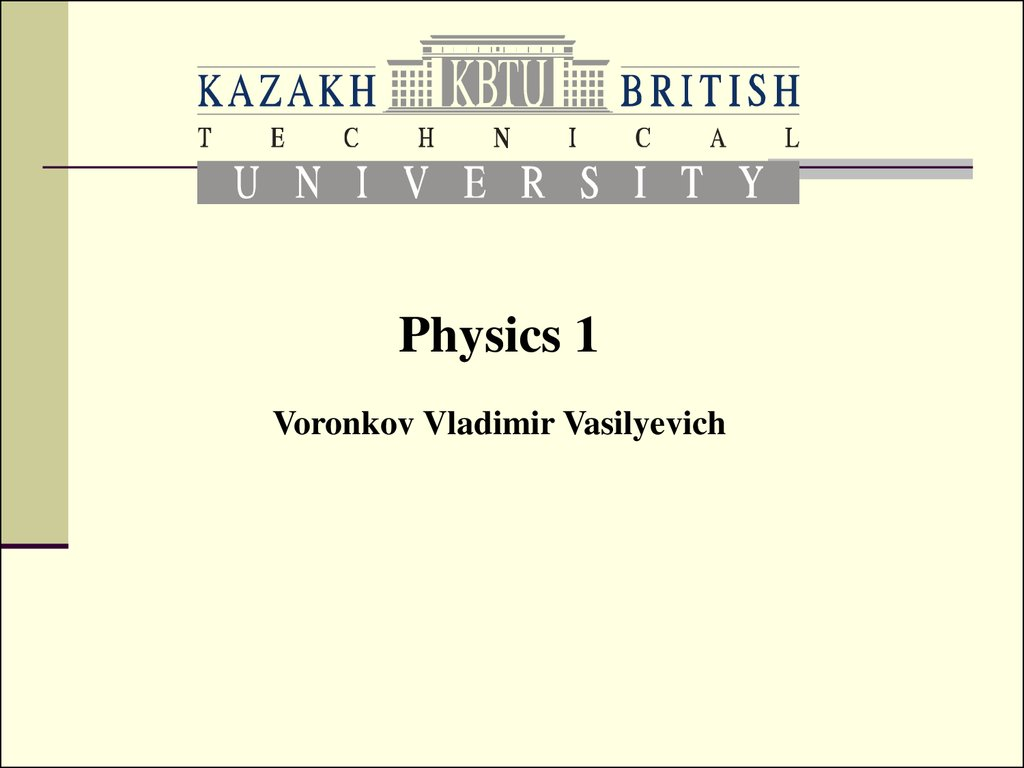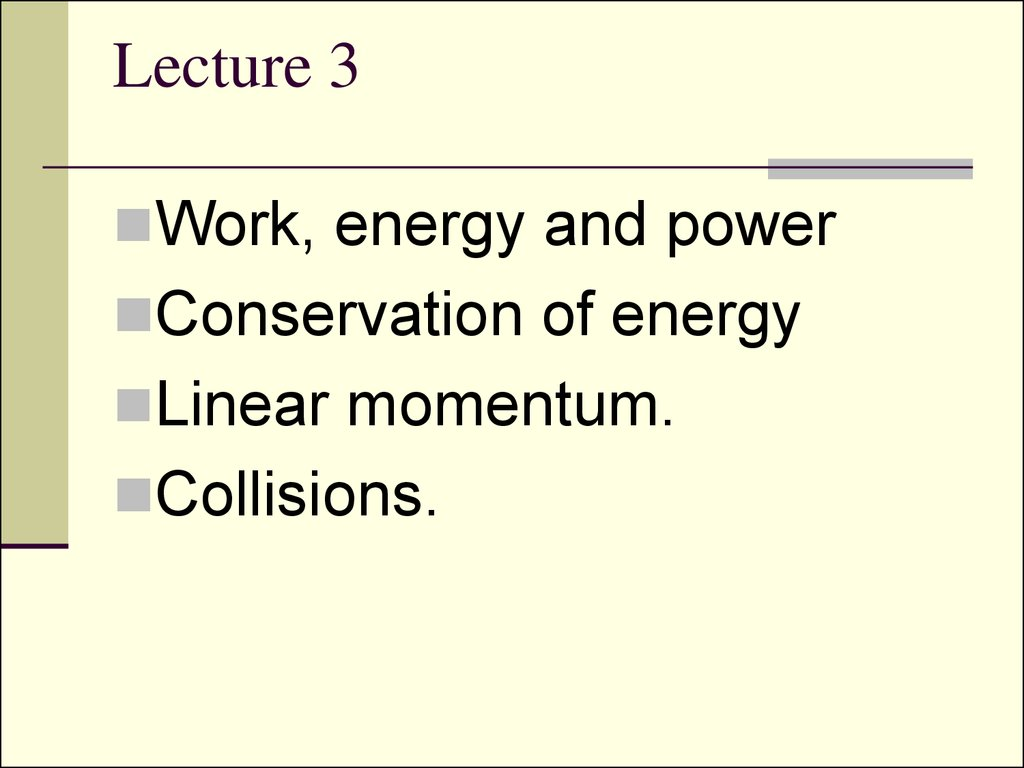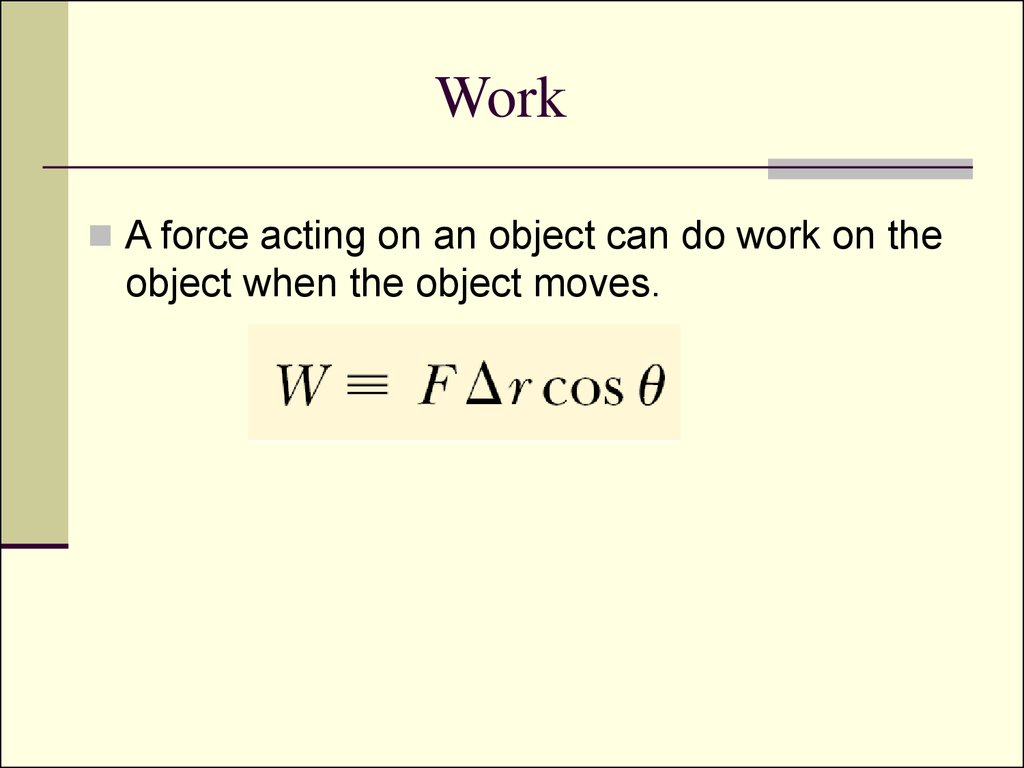# Work, energy and power. Conservation of energy. Linear momentum. Collisions

Physics 1

## 2. Lecture 3

Work, energy and power
Conservation of energy
Linear momentum.
Collisions.

## 3. Work

A force acting on an object can do work on the
object when the object moves.

## 4.

When an object is displaced on a frictionless,
horizontal surface, the normal force n and the
gravitational force mg do no work on the object. In
the situation shown here, F is the only force doing
work on the object.

## 5. Work Units

Work is a scalar quantity, and its
units are force multiplied by length.
Therefore, the SI unit of work is the
newton • meter (N • m). This
combination of units is used so
frequently that it has been given a
name of its own: the joule ( J).

## 8. Work done by a spring

If the spring is either stretched or compressed
a small distance from its unstretched
(equilibrium) configuration, it exerts on the
block a force that can be expressed as

## 11. Work of a spring

So the work done by a spring from one
arbitrary position to another is:

## 12. Kinetic energy

Work is a mechanism for transferring energy into a
system. One of the possible outcomes of doing work
on a system is that the system changes its speed.
Let’s take a body and a force acting upon it:
Using Newton’s second law, we can substitute for the
magnitude of the net force
and then perform the following chain-rule
manipulations on the integrand:

## 13.

And finally:
This equation was generated for the specific
situation of one-dimensional motion, but it is a
general result. It tells us that the work done
by the net force on a particle of mass m is
equal to the difference between the initial and
final values of a quantity

## 14. Work-energy theorem:

In the case in which work is done on a system
and the only change in the system is in its
speed, the work done by the net force equals
the change in kinetic energy of the system.
This theorem is valid only for the case when
there is no friction.

## 15. Conservative and Nonconcervative Forces

Forces for which the work is independent of
the path are called conservative forces.
Forces for which the work depends on the
path are called nonconservative forces
The work done by a conservative force in
moving an object along any closed path is
zero.

## 16. Examples

Conservative Forces:
Spring
central forces
Gravity
Electrostatic forces
Nonconcervative Forces:
Various kinds of Friction

## 17.

Gravity is a conservative force:
An object of moves from point A
to point B on an inclined plane
under the intluence of gravity.
Gravity does positive (or
negative) work on the object as
it move down (or up) the plane.
The object now moves from
point A to point B by a different
path: a vertical motion from
point A to point C followed by a
horizontal movement from C to
B. The work done by gravity is
exactly the same as in part (a).

## 19. Power

Power P is the rate at which work is done:

## 20. Potential Energy

Potential energy is the energy possessed by
a system by virtue of position or condition.
We call the particular function U for any given
conservative force the potential energy for
that force.
Remember the minus in the formula above.

## 23. Conservation of mechanical energy

E = K + U(x) = ½ mv2 + U(x) is called
total mechanical energy
If a system is
isolated (no energy transfer across its boundaries)
having no nonconservative forces within
then the mechanical energy of such a system
is constant.

## 24. Linear momentum

Let’s consider two interacting particles:
and their accelerations are:
using definition of acceleration:
masses are constant:

## 25.

So the total sum of quantities mv for an
isolated system is conserved – independent
of time.
This quantity is called linear momentum.

## 26.

General form for Newton’s second law:
It means that the time rate of change of the
linear momentum of a particle is equal to the
net for force acting on the particle.
The kinetic energy of an object can also be
expressed in terms of the momentum:

## 27. The law of linear momentum conservation

The sum of the linear momenta
of an isolated system of objects
is a constant, no matter what
forces act between the objects
making up the system.

## 28. Impulse-momentum theorem

The impulse of the force F acting on a particle
equals the change in the momentum of the
particle.
Quantity
is called the impulse of the
force F.

## 29. Collisions

Let’s study the following types of collisions:
1. Perfectly elastic collisions:
1.
2.
no mass transfer from one object to another
Kinetic energy conserves (all the kinetic
energy before collision goes to the kinetic
energy after collision)
2. Perfectly inelastic collisions: two objects
merge into one. Maximum kinetic loss.

## 30. Perfectly elastic collisions

We can write momentum and energy conservation
equations:
(1)=>
(2)=>
(4)/(3):
(1)
(2)
(3)
(4)
(5)

## 31.

Denoting
We can obtain from (5)
Here Ui and Uf are initial and final relative velocities.
So the last equation says that when the collision is
elastic, the relative velocity of the colliding objects
changes sign but does not change magnitude.

Work,Energy
Power
Linear momentum
W,E
P
p
J=N*m=kg*m2/s2
J/s=kg*m2/s3
kg*m/s